# How to Add in Excel – Master The Basics of Excel

Posted April 1, 2019 by Sheraz Ali in Microsoft ExcelThere are many ways of adding two or more numbers in excel.

You will have to get familiar with crafting formulas, which isn’t that complex. Learning how to construct formulae will not only teach you how to add in excel but also multiply, divide and subtract. In this tutorial, however, our main focus will be on adding two or more numbers.

To start off, let’s get a grasp of the basics.

### How does Excel define a Formula?

In Microsoft Excel, everything that starts with a = sign is perceived as a formula. So, it’s important to start your formulae with this sign, otherwise, Excel will register as a random stream of data.

A formula is comprised of an operator and an operand.

For instance, if you want to calculate a 10% discount from a 100\$ worth bag, the 20\$ and 10\$ are the operands and the multiply sign (*) the operator.

The final formula that you’ll have to input in excel will come out something like this.

=100\$(price)*.10(discount).

You simply have to type the above formula in one of the boxes in an Excel spreadsheet if you want to compute the following discount.

### Method 1: SUM Formula

1.In your excel spreadsheet, below the vertical column you want to add, insert the sum formula like this: =SUM(.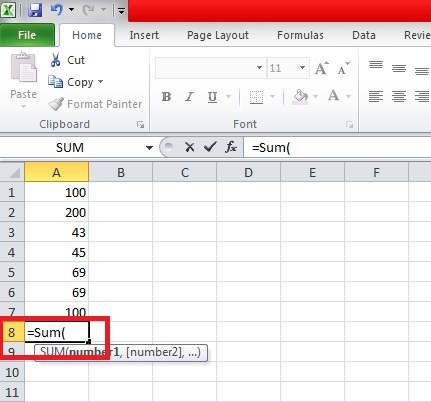2.Now, select the cells with the numbers you want to add.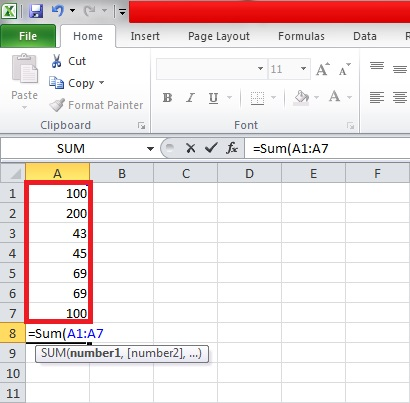3.Close the SUM Bracket and hit Enter.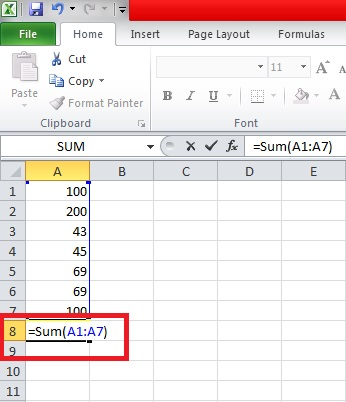4.All of your numbers will add up after hitting ENTER.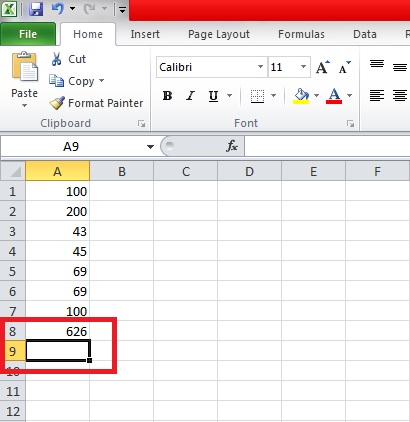### Method 2: Add Two or More Numbers in a Complete Row or Column of Cells

1.Write the same Formula =SUM(  in a third column and select the cells you want to add.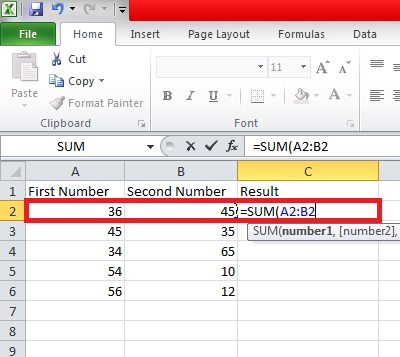2.Close the bracket of the formula: =Sum ( ) and hit Enter. The numbers in the selected cells will add up.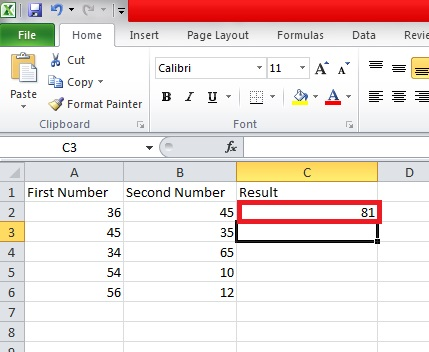3.To add the numbers in the remaining columns just select the first added column and extend it to the rest of the cells.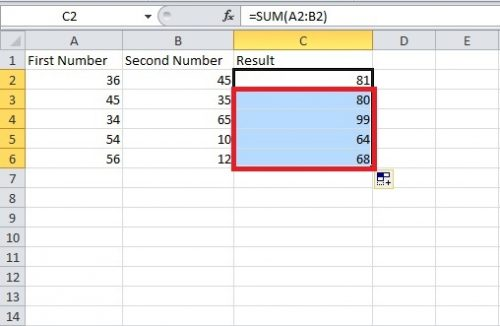4.Hit Enter and your numbers will add automatically.

That’s how simple it is to add in excel. It doesn’t take a minute. In my opinion, the method number two is the fasted way of performing addition on any version of Excel.

If I were you, I would go with it.
There are other tedious methods of accomplishing this but I have refrained from shedding a light on them because they’re in applicable in a lot of situations.

If you have any questions, please drop them below and I’ll personally get back to you and help you understand the basics of excel in any way I can.

You can also check related articles for further guidance.

Excel: Convert columns to rows

Create Hyperlink to another Worksheet

### Frequently Asked Questions

Can I perform these methods of addition in older versions of Microsoft excel? Are there any changes?

You can use these methods in older versions of excel, without any hassles. The methodology is quite similar and doesn’t deviate a lot.

Can I subtract cells excel in a similar way?

Yes, you can but it might require some maneuvering and changing up things. To understand how to subtract in excel, please read our tutorial on that for guidance.

### About Sheraz Ali

An established copywriter, with a longstanding experience in a vast array of industries, including but not limited to spirituality, technology, cannabis and travel.
View more articles by Sheraz Ali

#### The Conversation

Follow the reactions below and share your own thoughts.